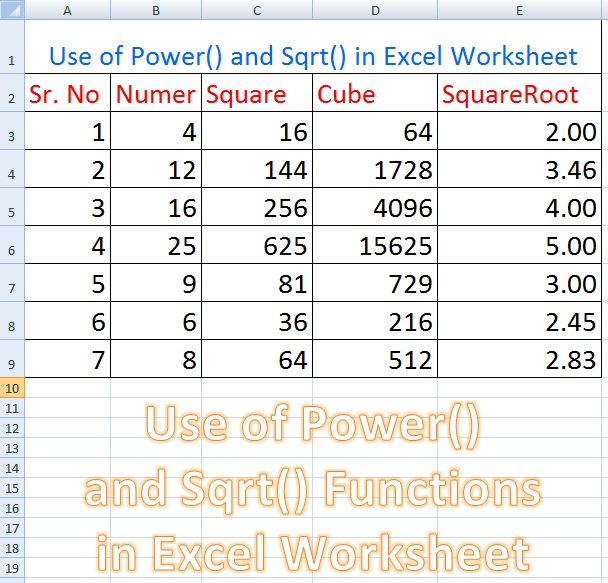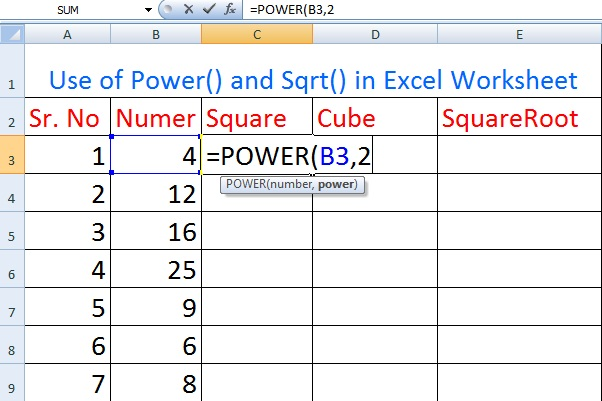# Use of Power and Sqrt Function in Excel Worksheet

•
•
•
•
•
•
•
•
•
•
Today, we will discuss the use of POWER( ) function and SQRT( ) function in Microsoft Excel Worksheets. We will prepare  a worksheet to practically learn the use of Power () function and Sqrt() function

as shown in the following figure:Use of POWER( ) function and SQRT( ) function in Microsoft Excel Worksheets

### The Use of POWER( ) function in MS Excel Worksheet

POWER function may be used to raise a number to a certain power. For example, to calculate number 2 raise to the power of 3, we will type the power function as:
=POWER(2,3)
which will return the result 8. If the number is in cell B3, we will type the power function as follows:
=POWER(B2,3)

### Steps To Create an Excel Worksheet To Demonstrate Use of Power() and Sqrt() Function

1. Start Microsoft Excel with a new blank workbook.
2. Type the data as shown below in the first worksheet.
3. Perform formatting in Excel worksheet as shown in the following figure.
4. Type data in Column A and Column B of worksheet.
5. In cell C3 of column C , we will type a formula using Power() function to calculate Square of given number.
6. Type = in cell with reference C3.
7. Type POWER(B3,2) and press Enter.
8. Since we have entered number 4 in B3 cell so Power(B3,2) will return 16 in cell C3.The Use of POWER( ) function in MS Excel Workshee

### The Use of SQRT( ) function in MS Excel Worksheet

The Excel function SQRT is used to calculate the square root of any number given as its argument.
For example, if you use wish to calculate square root of 4, you will type SQRT function as follows:
=SQRT(4)
which will give 2 as a result.

9.   In cell D3 of column D , we will type a formula using Power() function to calculate Cube of given number.
10. Type = in cell with reference D3.
11. Type POWER(B3,3) and press Enter.
12. Since we have entered number 4 in B3 cell so Power(B3,3) will return 64 in cell D3.The Use of SQRT( ) function in MS Excel Worksheet
13. In cell with reference E3  , we will type a formula using Sqrt() function to calculate Square root of given number.
14. Type = in cell with reference E3.
15. Type SQRT(B3) and press Enter.
16. Since we have entered number 4 in B3 cell so SQRT(B3) will return 2 in cell E3.
We have completed the task of creating a worksheet in MS Excel using Power function and SQRT() function.